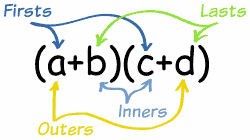# How do you foil (–7x – 11)(19x – 14)?

Aug 28, 2015

color(red)((–7x–11)(19x–14) = -133x^2 - 111x + 154)

$\text{FOIL}$ stands for $\text{F}$irst, $\text{O}$uter, $\text{I}$nner, $\text{L}$ast.Your expression is (–7x–11)(19x–14).

So you take each product in turn and then add them up.

We multiply the $\text{F}$irst term in both parentheses together:

-7x×19x = -133x^2

Then the $\text{O}$uter terms

-7x×(-14) = 98x

Then the $\text{I}$nner terms

-11×19x= -209x

And finally the $\text{L}$ast terms.

-11×(-14)=154

Then we add them up to get our expression:

(–7x–11)(19x–14)= -133x^2+98x-209x+154

We combine like terms to get the answer:

(–7x–11)(19x–14) = -133x^2 - 111x + 154